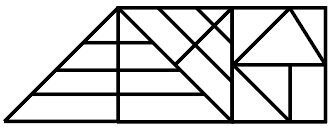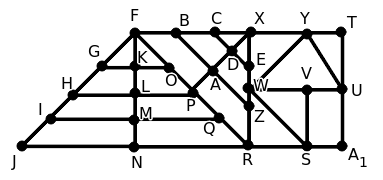Question 7

# How many triangles are there in the figure given below ?SolutionThe different triangles in the given figure are FKG, FKO, FGO, FLH, FLP, FHP, FMI, FMQ, FIQ, FNJ, FNR, FJR, XDC, XDE, XCE, XAB, XAZ, XBZ, XPF, XPR, XFR, XYW, YWU, YTU, VWS, WRS

$$\therefore\$$Number of triangles in the given figure = 26

Hence, the correct answer is Option C